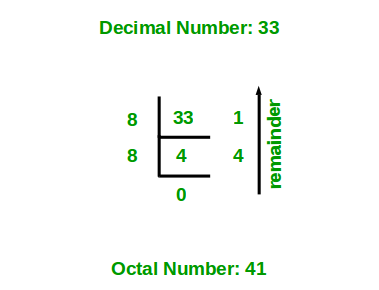# Program for Decimal to Octal Conversion

Given a decimal number as input, we need to write a program to convert the given decimal number into equivalent octal number. i.e convert the number with base value 10 to base value 8. The base value of a number system determines the number of digits used to represent a numeric value. For example, the binary number system uses two digits 0 and 1, octal number system uses 8 digits from 0-7 and decimal number system uses 10 digits 0-9 to represent any numeric value.

Examples:

```Input : 16
Output : 20

Input : 10
Output : 12

Input: 33
Output: 41
```

## Recommended: Please try your approach on {IDE} first, before moving on to the solution.

Algorithm:

1. Store the remainder when the number is divided by 8 in an array.
2. Divide the number by 8 now
3. Repeat the above two steps until the number is not equal to 0.
4. Print the array in reverse order now.

For Example:
If the given decimal number is 16.
Step 1: Remainder when 16 is divided by 8 is 0. Therefore, arr = 0.
Step 2: Divide 16 by 8. New number is 16/8 = 2.
Step 3: Remainder when 2 is divided by 8 is 2. Therefore, arr = 2.
Step 4: Divide 2 by 8. New number is 2/8 = 0.
Step 5: Since number becomes = 0. Stop repeating steps and print the array in reverse order. Therefore the equivalent octal number is 20.

Below diagram shows an example of converting the decimal number 33 to equivalent octal number.Below is the implementation of above idea.

## C/C++

 `// C++ program to convert a decimal ` `// number to octal number ` ` `  `#include ` `using` `namespace` `std; ` ` `  `// function to convert decimal to octal ` `void` `decToOctal(``int` `n) ` `{ ` `     `  `    ``// array to store octal number ` `    ``int` `octalNum; ` ` `  `    ``// counter for octal number array ` `    ``int` `i = 0; ` `    ``while` `(n != 0) { ` ` `  `        ``// storing remainder in octal array ` `        ``octalNum[i] = n % 8; ` `        ``n = n / 8; ` `        ``i++; ` `    ``} ` ` `  `    ``// printing octal number array in reverse order ` `    ``for` `(``int` `j = i - 1; j >= 0; j--) ` `        ``cout << octalNum[j]; ` `} ` ` `  `// Driver program to test above function ` `int` `main() ` `{ ` `    ``int` `n = 33; ` ` `  `    ``decToOctal(n); ` ` `  `    ``return` `0; ` `} `

## Java

 `// Java program to convert a decimal ` `// number to octal number ` `import` `java.io.*; ` ` `  `class` `GFG  ` `{ ` `    ``// Function to convert decimal to octal ` `    ``static` `void` `decToOctal(``int` `n) ` `    ``{ ` `        ``// array to store octal number ` `        ``int``[] octalNum = ``new` `int``[``100``]; ` ` `  `        ``// counter for octal number array ` `        ``int` `i = ``0``; ` `        ``while` `(n != ``0``)  ` `        ``{ ` `            ``// storing remainder in octal array ` `            ``octalNum[i] = n % ``8``; ` `            ``n = n / ``8``; ` `            ``i++; ` `        ``} ` ` `  `        ``// Printing octal number array in reverse order ` `        ``for` `(``int` `j = i - ``1``; j >= ``0``; j--) ` `            ``System.out.print(octalNum[j]); ` `    ``} ` `     `  `    ``// driver program ` `    ``public` `static` `void` `main (String[] args)  ` `    ``{ ` `        ``int` `n = ``33``; ` `        ``decToOctal(n);  ` `    ``} ` `} ` ` `  `// Contributed by Pramod Kumar `

## Python3

 `# Python3 program to convert  ` `# a decimal number to  ` `# octal number ` ` `  `# function to convert ` `# decimal to octal ` `def` `decToOctal(n): ` `     `  `    ``# array to store ` `    ``# octal number ` `    ``octalNum ``=` `[``0``] ``*` `100``; ` ` `  `    ``# counter for octal ` `    ``# number array ` `    ``i ``=` `0``; ` `    ``while` `(n !``=` `0``): ` ` `  `        ``# storing remainder ` `        ``# in octal array ` `        ``octalNum[i] ``=` `n ``%` `8``; ` `        ``n ``=` `int``(n ``/` `8``); ` `        ``i ``+``=` `1``; ` ` `  `    ``# printing octal number ` `    ``# array in reverse order ` `    ``for` `j ``in` `range``(i ``-` `1``, ``-``1``, ``-``1``): ` `        ``print``(octalNum[j], end ``=` `""); ` ` `  `# Driver Code ` `n ``=` `33``; ` ` `  `decToOctal(n); ` ` `  `# This code is contributed  ` `# by mits `

## C#

 `// C# program to convert a decimal ` `// number to octal number ` `using` `System; ` ` `  `class` `GFG { ` `     `  `    ``// Function to convert decimal to octal ` `    ``static` `void` `decToOctal(``int` `n) ` `    ``{ ` `         `  `        ``// array to store octal number ` `        ``int` `[]octalNum = ``new` `int``; ` ` `  `        ``// counter for octal number array ` `        ``int` `i = 0; ` `        ``while` `(n != 0)  ` `        ``{ ` `             `  `            ``// storing remainder in octal array ` `            ``octalNum[i] = n % 8; ` `            ``n = n / 8; ` `            ``i++; ` `        ``} ` ` `  `        ``// Printing octal number array in ` `        ``// reverse order ` `        ``for` `(``int` `j = i - 1; j >= 0; j--) ` `            ``Console.Write(octalNum[j]); ` `    ``} ` `     `  `    ``// driver program ` `    ``public` `static` `void` `Main ()  ` `    ``{ ` `        ``int` `n = 33; ` `         `  `        ``decToOctal(n);  ` `    ``} ` `} ` ` `  `// This code is contributed by nitin mittal. `

## PHP

 `= 0; ``\$j``--) ` `        ``echo` `\$octalNum``[``\$j``]; ` `} ` ` `  `// Driver Code ` `\$n` `= 33; ` ` `  `decToOctal(``\$n``); ` ` `  `// This code is contributed  ` `// by ajit ` `?> `

Output:

```41
```

This article is contributed by Harsh Agarwal. If you like GeeksforGeeks and would like to contribute, you can also write an article using contribute.geeksforgeeks.org or mail your article to contribute@geeksforgeeks.org. See your article appearing on the GeeksforGeeks main page and help other Geeks.My Personal Notes arrow_drop_up

1

Please write to us at contribute@geeksforgeeks.org to report any issue with the above content.